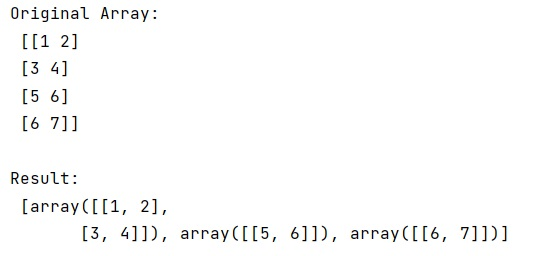# Partition array into N chunks with NumPy

Learn, how to Partition array into N chunks with NumPy in Python?
Submitted by Pranit Sharma, on January 11, 2023

NumPy is an abbreviated form of Numerical Python. It is used for different types of scientific operations in python. Numpy is a vast library in python which is used for almost every kind of scientific or mathematical operation. It is itself an array which is a collection of various methods and functions for processing the arrays.

## NumPy - Partitioning array into N chunks

Suppose we are given a NumPy array and we need to split it into N chunks.

For this purpose, we will use numpy.array_split() method. It split an array into multiple sub-arrays.

For an array of length L that should be split into n sections, it returns L % n sub-arrays of size : L//n + 1 and the rest of size L//n.

Let us understand with the help of an example,

## Python code for partitioning array into N chunks with NumPy

```# Import numpy
import numpy as np

# Creating an array
arr = np.array([[1,2],[3,4], [5,6], [6,7]])

# Display original array
print("Original Array:\n",arr,"\n")

# Splitting array into 3 parts
res = np.array_split(arr, 3)

# Display result
print("Result:\n",res)
```

Output:What's New (MCQs)

Top Interview Coding Problems/Challenges!

IncludeHelp's Blogs

Languages: » C » C++ » C++ STL » Java » Data Structure » C#.Net » Android » Kotlin » SQL
Web Technologies: » PHP » Python » JavaScript » CSS » Ajax » Node.js » Web programming/HTML
Solved programs: » C » C++ » DS » Java » C#
Aptitude que. & ans.: » C » C++ » Java » DBMS
Interview que. & ans.: » C » Embedded C » Java » SEO » HR
CS Subjects: » CS Basics » O.S. » Networks » DBMS » Embedded Systems » Cloud Computing
» Machine learning » CS Organizations » Linux » DOS
More: » Articles » Puzzles » News/Updates